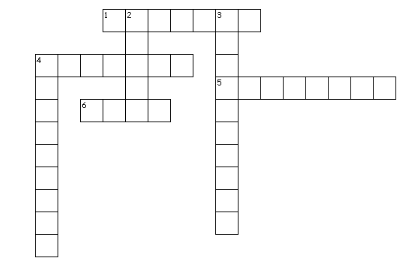# Comparing Quantities Worksheet for Class 7 Maths Chapter 7

In this page we have Comparing Quantities Worksheet for Class 7 Maths Chapter 7 . Hope you like them and do not forget to like , social share and comment at the end of the page.
Question 1
Fill in the blanks:-
i. While comparing two quantities their _________ should be same.
ii. if two ratios are equivalent then the four quantities are said to be in _____________
iii. Product of extremes = product of ______________ in a proportion.
iv. In a proportion a : b : : c : d, d is called the ___________________ proportional.
v. $Profit % = \frac {profit}{x} \times 100$ . x is ________
vi. The value of a machine depreciates 20% annually. If its present value is Rs. 20,000, its value after 2 years will be
vii. Percentages related to ____________ fractions are more than 100.
viii. The money that is borrowed is known as ___________________
ix. x% of y is y% of ___________
x. 23% + 47% + __________ = 100%
xi.  The ratio 3 : 8 is equal to _____%
xii. 0.05 is equivalent to ___%
Question 2
True or false:
i. A ratio get changed if both of its terms are multiplied by the same non-zero quantity.
ii. Profit % or Loss % is always calculated on selling price.
iii.  The price at which an article is sold is called the cost price.
iv. Percent means thousand.
v. In unitary method, we find the value of many units then find the value of one unit.
vi. 3 : 5 means 60%
vii. The ratio 5 : 12 is greater than 3 : 5
viii. The amount paid on   P at R% p.a. after T years is PRT/100
ix. S.P. = C.P. + Loss.
x. The ratio 2 : 3 and 3 :2 are equivalent.
xi. 120 % of 50 km is 60 km
xii. 15 kg is 30% of 50 kg
Question  3.
Find the ratio of
a. 2m 7cm : 36cm
b. 3kg 250g : 5kg
Question 4.
Are 25,15,6,5 in proportion ?
Question 5.
In an examination 86% of candidates passed and 70 failed. How many candidates appeared?
Question 6.
Find 10% more than Rs. 90.

Question 7.
x% of  25/2  is 150. What is value of x?
Question 8.
What is the Interest on Rs  12000 for 1 month at the rate of 10 % per annum
Question 9.
What percent of  2/7 is 1/35
Question 10.
If 40% of a no is 256, what is 25% of that number.
Question 111. Per cent is derived from Latin word ‘per centum’ meaning ‘per ____’.
4. The difference SP - CP
5. Amount = Principal + ______
6. The difference CP - SP
Down
2. the ______ must be the same to compare two quantities
3. 1:2  and 2:4 are _____ ratio
4. The money you borrow is known as _____`

1.
i. unit
ii. proportion
iii. middle term
iv.  Fourth
v. CP
vi. Rs 12000
vii. improper
viii. principal
ix. x
x. 30%
xi. 37.5%
xii 5 %
2.
i. False
ii. false
iii. false
iv. false
v. false
vi. true
vii. false
viii. false
ix false
x. false
xi. True
xii. True

3.
a. 13: 4
b. 13:20

4.  Not proprtion
5. 500
6. 99
7.  1200
8.  100
9.10%
10. 160
11
a. hundred
b.units
c.equivalent
d. principal, profit
e. interest
f. loss

• Worksheets